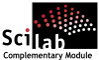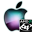ATOMS : Number details

# Number

Number theory related algorithmsDetails
Version
0.2
A more recent valid version with binaries for Scilab 5.2 exists: 1.2
Author
Michael Baudin
Owner Organization
Consortium Scilab - DIGITEO
Maintainer
Michael BAUDIN
Category
Creation Date
November 24, 2009
Source created on
Scilab 5.2.x
Binaries available on
Scilab 5.2.x:Windows 64-bitWindows 32-bitLinux 64-bitLinux 32-bitmacOS
Install command
`--> atomsInstall("number")`
Report a bugDescription
```            The goal of this toolbox is to provide several Number theory related
algorithms.
The following is a list of the current features:
* primality test with
* fast mass division
* various uses of congruences
* probable primality test with Miller-Rabin test
* pseudo-prime test
* greatest common divisor with
* naive recursive or iterative Euclid's algorithm
* brute force search for divisors
* binary recursive or iterative algorithm
* linear equation solver ax+by=d by extended Euclid's algorithm
* least common multiple
* list of primes lower than by either naive or fast Erathostene's sieve
* factoring into primes by
* either naive or fast trial division
* Fermat's difference of squares
* Pollard-rho
* solver for linear equation ax = b mod m
* modular inverse
* power of an integer modulo m
* decompose an integer on a given basis
* table of 1000 first primes
* Fermat, Mersenne, Carmichael numbers

The toolbox is based on macros.
The implementation uses Scilab's double precision integers,
therefore is limited to integers in the [-2^52,2^52] interval.
Many features are missing so that the current state is more a "toy" toolbox.

```Files (2)Source code archive
```Number sources
```OS-independent binary for Scilab 5.2.x
```Binary version
Automatically generated by the atoms compilation chain
```News (0)Comments (0)Leave a comment
Login withEmail notifications